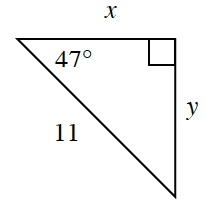### Home > CCG > Chapter 5 > Lesson 5.1.1 > Problem5-7

5-7.You now have multiple trig tools to find missing side lengths of triangles. For the triangle at right, find the values of $x$ and $y$. Your Triangle Toolkit might help. Which tools did you use?

Use trig ratios. The hypotenuse is $11$.
Is $x$ opposite from or adjacent to the $47^\circ$ angle.
What about $y$?